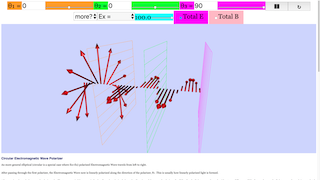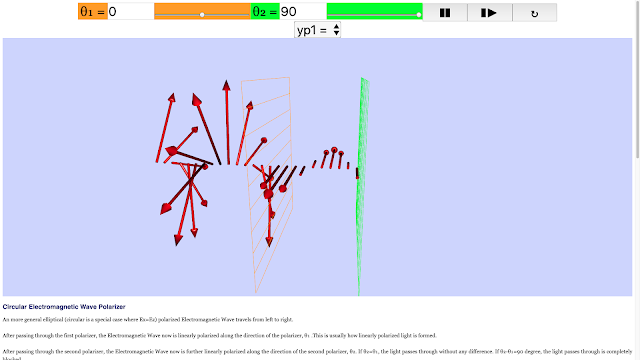Introduction

## Circular Electromagnetic Wave Polarizer

An more general elliptical (circular is a special case where Ex=Ez) polarized Electromagnetic Wave travels from left to right.

After passing through the first polarizer, the Electromagnetic Wave now is linearly polarized along the direction of the polarizer, θ₁ .This is usually how linearly polarized light is formed.

After passing through the second polarizer, the Electromagnetic Wave now is further linearly polarized along the direction of the second polarizer, θ₂. If θ₂=θ₁, the light passes through without any difference. If θ₂-θ₁=90 degree, the light passes through is completely blocked.

After passing through the third polarizer,the Electromagnetic Wave now is further linearly polarized along the direction of the third polarizer, θ₃. If θ₃ = θ₂= θ₁,the linearly polarized light passes through without any difference. If θ₃-θ₂=90 degree and θ₂-θ₁=0 degree, light inbetween polarizer 2 and 3 is unchanged, still linearly polarized, but light after polarizer 3 is completely blocked.

## Visualization

Electric Field is represented as Red color segments

Magnetic Field is represented as Blue color segments

Polarizer 1 is Orange color

Polarizer 2 is Green color

Polarizer 3 is Magenta color

## Controls

You can modify:

θ₁ is the angle of the first polarizer

θ₂ is the angle of the second polarizer

θ₃ is the angle of the third polarizer

under the combobox

yp₁ is the position of the first polarizer

yp₂ is the position of the second polarizer

yp₃ is the position of the third polarizer

under more? it is possible to explore these other more advance variables

Ex: electric field magnitude in x direction

Ez: electric field magnitude in z direction

phase: the phase difference between E and B

vy: wave velocity. which imply Ez/Bx=vy, where Bx is magnetic field magnitude

T :period of the wave. which imply wavelength(lamda)=vy*T

wavelength: the length of one cycle of the wave

Please notice that E/B field are in phase when electromagnetic is travel in vacuum.

### Translations

Code Language Translator Run### Software Requirements

SoftwareRequirements

 Android iOS Windows MacOS with best with Chrome Chrome Chrome Chrome support full-screen? Yes. Chrome/Opera No. Firefox/ Samsung Internet Not yet Yes Yes cannot work on some mobile browser that don't understand JavaScript such as..... cannot work on Internet Explorer 9 and below

### CreditsFremont Teng; Fu-Kwun Hwang; lookang

## Circular Electromagnetic Wave Polarizer JavaScript Simulation Applet HTML 5

Circular Electromagnetic Wave 2 Polarizer JavaScript Simulation Applet HTML 5A more general elliptical (circular is a special case where Ex=Ez) polarized Electromagnetic Wave travels from left to right. After passing through the first polarizer, the Electromagnetic Wave now is linearly polarized along the direction of the polarizer, θ₁ .This is usually how linearly polarized light is formed.After passing through the second polarizer, the Electromagnetic Wave now is further linearly polarized along the direction of the second polarizer, θ₂. If θ₂=θ₁, the light passes through without any differenceA more general elliptical (circular is a special case where Ex=Ez) polarized Electromagnetic Wave travels from left to right. After passing through the first polarizer, the Electromagnetic Wave now is linearly polarized along the direction of the polarizer, θ₁ .This is usually how linearly polarized light is formed.After passing through the second polarizer, the Electromagnetic Wave now is further linearly polarized along the direction of the second polarizer, θ₂. If θ₂-θ₁=90 degree, the light passes through is completely blocked.

## Circular Electromagnetic Wave Polarizer

A more general elliptical (circular is a special case where Ex=Ez) polarized Electromagnetic Wave travels from left to right.

After passing through the first polarizer, the Electromagnetic Wave now is linearly polarized along the direction of the polarizer, θ₁ .This is usually how linearly polarized light is formed.

After passing through the second polarizer, the Electromagnetic Wave now is further linearly polarized along the direction of the second polarizer, θ₂. If θ₂=θ₁, the light passes through without any difference. If θ₂-θ₁=90 degree, the light passes through is completely blocked.

## Visualization

Electric Field is represented as Red color segments

Magnetic Field is represented as Blue color segments

Polarizer 1 is Orange color

Polarizer 2 is Green color

## Controls

You can modify:

θ₁ is the angle of the first polarizer

θ₂ is the angle of the second polarizer

under the combobox

yp₁ is the position of the first polarizer

yp₂ is the position of the second polarizer

under more? it is possible to explore these other more advance variables

Ex: electric field magnitude in x direction

Ez: electric field magnitude in z direction

phase: the phase difference between E and B

vy: wave velocity. which imply Ez/Bx=vy, where Bx is magnetic field magnitude

T :period of the wave. which imply wavelength(lamda)=vy*T

Please notice that E/B field are in phase when electromagnetic is travel in vacuum.

### Instructions on using Simulation Applet

#### Combo Boxes

Selecting the Option will show you the sliders to change accordingly.

The most notable option would be 'choose components',
where you can select which of the fields to be visible.

#### Play, Pause and Reset Buttons

These two buttons will enable you to Play/Pause and Reset accordingly.

### end faq

Rating 5.00 (1 Vote)